## Quiz 12 : The Supply of and Demand for Productive ResourcesLooking for Economics Homework Help?# Quiz 12 : The Supply of and Demand for Productive Resources

A producer needs different factors of production, like land, labor, capital, and machinery, to produce different goods and services. The demand of these factors of production exists because there is a demand for goods and services they help produce. Demand for factors of production is a derived demand, because the consumer derives it from the production of goods and services, and their demand in the market. Employment of resources are inversely related to their price, because as the price of these resources increase, the cost of production increases and the producer will move to a lower priced substitute available in the market. On the other hand, if the price of resources is high, it leads to increase in the price of goods and services, and the consumer will move to low priced products. Therefore, the demand for factors of production and price are inversely related.

A firm's decision to hire depends upon the marginal benefit it gets from hiring an additional unit of factor of production and the marginal cost of hiring the additional unit. The marginal benefit of hiring is measured by the marginal revenue productivity (MRP), which decreases as a firm hires additional units of factor. MRP is the increase in total revenue of the firm by hiring an additional unit of factor, and it is equal to marginal product (MP) of factor and the price (P) of factor. That is,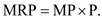A firm will continue hiring an additional unit of factor as long as MRP is greater than the marginal cost of hiring an additional unit of factor, and it will stop hiring when MRP becomes equal to the marginal cost. Assuming that factor inputs are perfectly divisible, the combination of skilled and unskilled labor that will be employed by a profit-maximizing firm will depend upon the MRP and the price of both skilled and unskilled labor. When the MRP weighted by price of skilled labor is equal to the MRP weighted by price of unskilled labor, it is said that a profit-maximizing firm is minimizing its cost. In other words, when the marginal product per dollar expenditure is equal for all resources, the firm has been said to minimize the cost of hiring multiple resources.

The short-run demand schedule of Compute-Accounting is shown as below.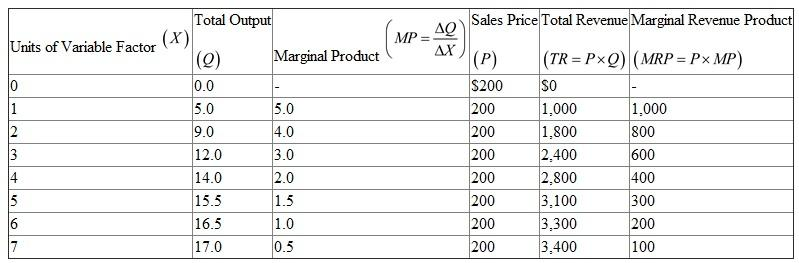a) A profit maximizing firm will expand employment of a variable resource until the marginal revenue product of the resource equates the cost of hiring it. Compute-Accounting will hire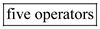at a weekly wage of \$250. Expanding employment further would make the marginal revenue product fall short of the weekly wage. b) Profit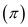of the firm is calculated as the difference between the total revenue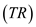and total cost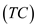. Total cost of the firm is the sum of variable cost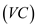and fixed cost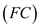. The total variable cost of hiring five operators is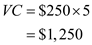With fixed cost of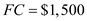per week, the total cost of the firm isThe total revenue of the firm at this level is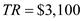. Calculate profit asThus, the profit of the firm is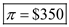. c) The new short-run demand schedule of Compute-Accounting is shown as below.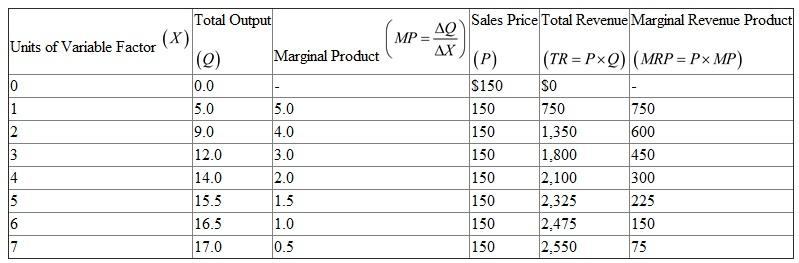Compute-Accounting will hire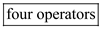at a weekly wage of \$250. Expanding employment further would make the marginal revenue product fall short of the weekly wage. The total variable cost of hiring four operators is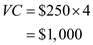With fixed cost ofper week, the total cost of the firm isThe total revenue of the firm at this level is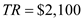. Calculate profit asAt lower price the firm incurs economic loss. Price is sufficient to cover average variable cost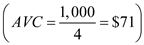but not average total cost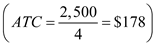. The firm, therefore, will operate in the short-run but will go out of business in the long-run unless the market price rises.